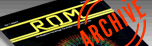`ROM MAGAZINE ISSUE 5 — APRIL/MAY 1984 / PAGE 57`

 SPEEDING UP YOUR JOYSTICK By BOB COCKROFT      Because the Atari Basic Language is so slow, any method to make programs operate faster can be quite useful. Joystick commands are an area which technique can minimize some of the languages weaknesses.     The first step is to set a variable equal to the stick position. ST=STICK(0)     Next determine whether the stick is pressed in the direction of the vertical(y) or horizontal (x) axis. SY=(ST=13)-(ST=14) SX=(ST=7)-(ST=11)      For example, if the stick is pushed in the direction which causes it to register a '7', SX will equal 1. Conversely if the stick registers an '11' SX will equal -1. In this manner, variables SY and SX will have provided a program with a more efficient means of registering joystick's position.     The following is a program that applies the principles mentioned to create a simple screen painter. The only significant difference from the example above is that this program provides for diagonal movement. 10 REM * JOYSTICK SUBROUTINE * 12 X=50:Y=50 20 GRAPHICS 8:COLOR 1:SETCOLOR 2,16,1:POKE 752,1 25 ? "        Press button to erase" 30 ST=STICK(0) 40 SY=(ST=13 OR ST=9 OR ST=5)-(ST=14 OR ST=10 OR ST=6) 50 SX=(ST=7 OR ST=6 OR ST=5)-(ST=11 OR ST=10 OR ST=9) 60 Y=Y+SY:X=X+SX 70 PLOT X,Y 75 IF STRIG(0)=0 THEN COLOR 0:GOTO 30 77 COLOR 1 80 GOTO 30